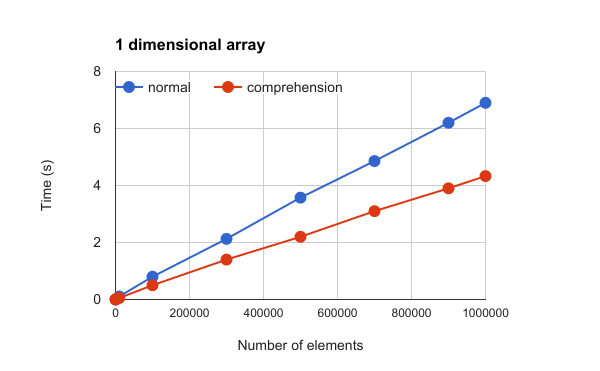# Description

There are a limit executing time for Google Apps Script (GAS). It’s 6 minutes. So users have to pay attention to the process cost of the script. GAS can use JavaScript 1.7. This means to be able to be used comprehension for GAS.

In this report, the process cost for the comprehension has been investigated. The normal for loop was used as the competitor. As a result, it was found that the comprehension can be used one of methods for reducing the process cost. For 1 dimensional array, the process cost of comprehension is about 60% lower than that of the normal one. For 2 dimensional array, the process cost of comprehension is about 50% lower than that of the normal one. Each data is the average for 10 times measurements.# Sample Script

As a sample, 1 dimensional array and 2 dimensional array were used. The continuous number was imported to 1 dimensional array. For 2 dimensional array, the number of 1 to 100 was imported each element. As the calculation for measuring the process cost, when the element was multiples of 5, it was retrieved. These samples are GAS.

## For 1D

function make1dAr(row){
var ar = [];
for (var i=0; i<row; i++) {
ar[i] = i + 1;
}
return ar;
}

function comprehension1d(ar){
return [i for each (i in ar) if(i % 5 == 0)];
}

function normal1d(ar){
var result = [];
for (var i=0; i<ar.length; i++) {
if (ar[i] % 5 == 0) {
result.push(ar[i]);
}
}
return result;
}

function main1d() {
var data = make1dAr(1000000);

var start_P_time1 = Date.now();
var res = normal1d(data);
var end_P_time1 = Date.now();
Logger.log((end_P_time1 - start_P_time1)/1000 + ' [s]')

var start_P_time1 = Date.now();
var res = comprehension1d(data);
var end_P_time1 = Date.now();
Logger.log((end_P_time1 - start_P_time1)/1000 + ' [s]')
}


## For 2D

function make2dAr(row){
var ar1 = [];
for (var i=0; i<row; i++) {
var ar2 = [];
for (var j=0; j<100; j++) {
ar2[j] = j + 1;
}
ar1[i] = ar2;
}
return ar1;
}

function comprehension2d(ar){
return [[j for each (j in i) if(j % 5 == 0)] for each (i in ar)];
}

function normal2d(ar){
var ar1 = [];
for (var i=0; i<ar.length; i++) {
var ar2 = [];
for (var j=0; j<ar[i].length; j++) {
if (ar[i][j] % 5 == 0) {
ar2.push(ar[i][j]);
}
}
ar1.push(ar2)
}
return ar1;
}

function main2d() {
var data = make2dAr(10000);

var start_P_time1 = Date.now();
var res = normal2d(data);
var end_P_time1 = Date.now();
Logger.log((end_P_time1 - start_P_time1)/1000 + ' [s]')

var start_P_time1 = Date.now();
var res = comprehension2d(data);
var end_P_time1 = Date.now();
Logger.log((end_P_time1 - start_P_time1)/1000 + ' [s]')
}